# Oops...

This website is made for modern browsers. You are seeing this, because your browser is missing a feature or two. Please install the latest update or switch to a modern browser. See you soon.

# Domains

#domains

This how-to guide is focusing on domains and the various components and component compositions that host valuable functions related to domains. In mathematics, a domain refers to the input values of a function and the range refers to the values that this function can output. In Grasshopper, both terms are often used indistinctly; a range can be a domain or a defined series of numbers. Domains are specified as a domain of numeric range. Despite this vague mathematical description, domains in Grasshopper are well-defined as a data type.

Domains in Grasshopper have defined bounds that are represented by a lower and a upper value, like 0 and 1. Within the domain, all real numbers are possible that exist between the bounds. A domain is written as 0 To 1. Beside this data type Domain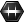Domain (Domain)
Params  >  Primitive  >  Domain
Contains a collection of numeric domains
, there is also the data type Domain²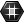Domain² (Domain²)
Params  >  Primitive  >  Domain²
Contains a collection of 2D number domains
that represents two domains in one object (this is different from having two domain-items in a list). The domains of Domain² are named u and v and this data construct is useful when working with surfaces.

### Construct Domains

The most official way to construct a domain is using the component Construct Domain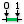Construct Domain (Dom)
Maths  >  Domain  >  Construct Domain
Create a numeric domain from two numeric extremes.
Inputs
Domain start (A)Start value of numeric domain
Domain end (B)End value of numeric domain
Outputs
Domain (I)Numeric domain between {A} and {B}
that converts two given numbers into bounds of a domain.

Another way is to use a PanelPanel
Params  >  Input  >  Panel
A panel for custom notes and text values
and to write the bounds with a To in the middle: 0 To 5. Whenever a component requests for a domain, this string format will be converted to one.

If a component requests a domain, but only a single number is provided, than this number becomes the upper bound and the lower bound is set to 0, as demonstrated with the DomainDomain (Domain)
Params  >  Primitive  >  Domain
Contains a collection of numeric domains
container.

The component Bounds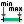Bounds (Bnd)
Maths  >  Domain  >  Bounds
Create a numeric domain which encompasses a list of numbers.
Inputs
Numbers (N)Numbers to include in Bounds
Outputs
Domain (I)Numeric Domain between the lowest and highest numbers in {N}
will take a list of numbers as input and analyzes it to set the smallest number as lower bound and the largest number as upper bound of a domain.

The component Consecutive Domains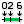Consecutive Domains (Consec)
Maths  >  Domain  >  Consecutive Domains
Create consecutive domains from a list of numbers
Inputs
Numbers (N)Numbers for consecutive domains
Outputs
Domains (D)Domains describing the spaces between the numbers
will take a list of numbers as input and creates multiple domains from it. By default, the value of each item relates to the numeric range of each domain, which could also be expressed as a step size between the domains.

If we set input A of Consecutive DomainsConsecutive Domains (Consec)
Maths  >  Domain  >  Consecutive Domains
Create consecutive domains from a list of numbers
Inputs
Numbers (N)Numbers for consecutive domains
Outputs
Domains (D)Domains describing the spaces between the numbers
to False, then each value will represent the upper bound of the current and the lower bound of the following domain.

To construct the data type Domain², there are two possibilities. The first one is to use Construct Domain²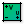Construct Domain² (Dom²Num)
Maths  >  Domain  >  Construct Domain²
Create a two-dimensinal domain from four numbers.
Inputs
U min (U0)Lower limit of domain in {u} direction
U max (U1)Upper limit of domain in {u} direction
V min (V0)Lower limit of domain in {v} direction
V max (V1)Upper limit of domain in {v} direction
Outputs
2D Domain (I²)Two dimensional numeric domain of {u} and {v}
, which constructs a Domain² from four numbers; the lower and upper bounds of each domain.

The second possibility is to use Construct Domain²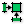Construct Domain² (Dom²)
Maths  >  Domain  >  Construct Domain²
Create a two-dimensional domain from two simple domains.
Inputs
Domain U (U)Domain in {u} direction
Domain V (V)Domain in {v} direction
Outputs
2D Domain (I²)Two dimensional numeric domain of {u} and {v}
, which takes two Domain-objects and converts them into a Domain².

### Deconstruct domains

Of course, if domains can be constructed, they can also be deconstructed and Deconstruct Domain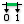Deconstruct Domain (DeDomain)
Maths  >  Domain  >  Deconstruct Domain
Deconstruct a numeric domain into its component parts.
Inputs
Domain (I)Base domain
Outputs
Start (S)Start of domain
End (E)End of domain
does this for the data type Domain. At the outputs, we get the lower and upper bound.

For the data type Domain², there are again two possibilities: The component Deconstruct Domain²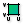Deconstruct Domain² (DeDom2Num)
Maths  >  Domain  >  Deconstruct Domain²
Deconstruct a two-dimensional domain into four numbers
Inputs
Domain (I)Base domain
Outputs
U min (U0)Lower limit of domain in {u} direction
U max (U1)Upper limit of domain in {u} direction
V min (V0)Lower limit of domain in {v} direction
V max (V1)Upper limit of domain in {v} direction
deconstructs the u and v domain into four numbers (lower and upper bounds).

The alternative is Deconstruct Domain²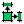Deconstruct Domain² (DeDom2)
Maths  >  Domain  >  Deconstruct Domain²
Deconstruct a two-dimensional domain into its component parts
Inputs
Domain (I)Base domain
Outputs
U component (U){u} component of domain
V component (V){v} component of domain
, which deconstructs the Domain² into its two separate domains at output U and V.

### Retrieve domains from geometric objects

Domains are often used in combination with geometric objects. At an object’s input, we can use Reparameterize to rewrite the actual domain into a normalized one (0 To 1). Then we relate our operations to the normalized domain and do not need to know the actual domain. This is useful, if the real bounds vary from shape to shape.

If we need to retrieve the real domain of a geometric shape, we can also do this. Curves have one direction and they translate into data type Domain. The component Curve Domain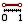Curve Domain (CrvDom)
Curve  >  Analysis  >  Curve Domain
Measure and set the curve domain
Inputs
Curve (C)Curve to measure/modify
Domain (D)Optional domain, if omitted the curve will not be modified.
Outputs
Curve (C)Curve with new domain.
Domain (D)Domain of original curve.
will retrieve the domain of a curve. Also, inputs that request a domain, but get a curve instead, will translate the curve into a domain, like DomainDomain (Domain)
Params  >  Primitive  >  Domain
Contains a collection of numeric domains
does.
In rare cases, because of type conversion, the result of Curve DomainCurve Domain (CrvDom)
Curve  >  Analysis  >  Curve Domain
Measure and set the curve domain
Inputs
Curve (C)Curve to measure/modify
Domain (D)Optional domain, if omitted the curve will not be modified.
Outputs
Curve (C)Curve with new domain.
Domain (D)Domain of original curve.
and DomainDomain (Domain)
Params  >  Primitive  >  Domain
Contains a collection of numeric domains
differs. Read Curve and surface parameters for an explanation.
In rare cases, because of type conversion, the result of Curve DomainCurve Domain (CrvDom)
Curve  >  Analysis  >  Curve Domain
Measure and set the curve domain
Inputs
Curve (C)Curve to measure/modify
Domain (D)Optional domain, if omitted the curve will not be modified.
Outputs
Curve (C)Curve with new domain.
Domain (D)Domain of original curve.
and DomainDomain (Domain)
Params  >  Primitive  >  Domain
Contains a collection of numeric domains
differs. Read Curve and surface parameters for an explanation.

A surface has two directions (u and v) and translates to data type Domain². For retrieving, we can use a Domain²Domain² (Domain²)
Params  >  Primitive  >  Domain²
Contains a collection of 2D number domains
container, because components that request for a Domain² will do the conversion automatically. Thus, we could also use the deconstruct-domain components to extract the two domains and the bounds.

If we have a cloud of points, we can use Bounds 2D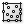Bounds 2D (Bnd)
Maths  >  Domain  >  Bounds 2D
Create a numeric two-dimensional domain which encompasses a list of coordinates.
Inputs
Coordinates (C)Two dimensional coordinates to include in Bounds
Outputs
Domain (I)Numeric two-dimensional domain between the lowest and highest numbers in {N.x ; N.y}
to create a Domain² from the two-dimensional coordinates (x and y) of the points. The z-coordinates are neglected. If we would like to do this for all three coordinates, we can use Deconstruct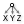Deconstruct (pDecon)
Vector  >  Point  >  Deconstruct
Deconstruct a point into its component parts.
Inputs
Point (P)Input point
Outputs
X component (X)Point {x} component
Y component (Y)Point {y} component
Z component (Z)Point {z} component
on the list of points and then use BoundsBounds (Bnd)
Maths  >  Domain  >  Bounds
Create a numeric domain which encompasses a list of numbers.
Inputs
Numbers (N)Numbers to include in Bounds
Outputs
Domain (I)Numeric Domain between the lowest and highest numbers in {N}
for each coordinate.

### Divide domains

Because domains host a range of numbers, we can also subdivide them into smaller units. The component Divide Domain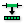Divide Domain (Div)
Maths  >  Domain  >  Divide Domain
Divide a domain into equal segments.
Inputs
Domain (I)Base domain
Count (C)Number of segments
Outputs
Segments (S)Division segments
divides domains into C smaller domains.

The component Divide Domain²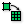Divide Domain² (Divide)
Maths  >  Domain  >  Divide Domain²
Divides a two-dimensional domain into equal segments.
Inputs
Domain (I)Base domain
U Count (U)Number of segments in {u} direction
V Count (V)Number of segments in {v} direction
Outputs
Segments (S)Individual segments
does the same for data type Domain² and the number of segments in each direction can be set individually. The division relates to a grid layout, so the number of domains created is the product U and V.

The operation Divide Domain²Divide Domain² (Divide)
Maths  >  Domain  >  Divide Domain²
Divides a two-dimensional domain into equal segments.
Inputs
Domain (I)Base domain
U Count (U)Number of segments in {u} direction
V Count (V)Number of segments in {v} direction
Outputs
Segments (S)Individual segments
is useful in combination with Isotrim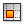Isotrim (SubSrf)
Surface  >  Util  >  Isotrim
Extract an isoparametric subset of a surface.
Inputs
Surface (S)Base surface
Domain (D)Domain of subset
Outputs
Surface (S)Subset of base surface
: Divide Domain² automatically converts a plugged in surface into a domain² and creates a subset of surface parameters. Then, Isotrim will slice the surface into smaller segments.

The component Range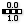Range (Range)
Sets  >  Sequence  >  Range
Create a range of numbers.
Inputs
Domain (D)Domain of numeric range
Steps (N)Number of steps
Outputs
Range (R)Range of numbers
will also divide a domain. But not into smaller segmented domains but in real numbers. Input N sets the number of steps that the domain is divided into.

### Remap a domain

The component Remap Numbers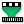Remap Numbers (ReMap)
Maths  >  Domain  >  Remap Numbers
Remap numbers into a new numeric domain
Inputs
Value (V)Value to remap
Source (S)Source domain
Target (T)Target domain
Outputs
Mapped (R)Remapped number
Clipped (C)Remapped and clipped number
will remap a given value V, which is part of the source domain S, to the correlating value in the target domain T. Given the target domain 0 To 1, we can use this component to normalize numbers. See how-to Curve and surface parameters for more about reparameterization.

### Check domains

To test a number for its relation to one or more domains, there are two components present in Grasshopper. Includes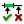Includes (Inc)
Maths  >  Domain  >  Includes
Test a numeric value to see if it is included in the domain
Inputs
Value (V)Value to test for inclusion
Domain (D)Domain to test with
Outputs
Includes (I)True if the value is included in the domain
Deviation (D)Distance between the value and the nearest value inside the domain
will check if a given value V is part of the domain D.

In case that the number is not part of the domain, output D will state the distance between the given number and the nearest bound of the domain.

The component Find Domain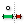Find Domain (FDom)
Maths  >  Domain  >  Find Domain
Find the first domain that contains a specific value
Inputs
Domains (D)Collection of domains to search
Number (N)Number to test
Strict (S)Strict comparison, if true then the value must be on the interior of a domain
Outputs
Index (I)Index of first domain that includes the specified value
Neighbour (N)Index of domain that is closest to the specified value
is similar, but here we provide a collection of domains at input D and the function will find the domain in which the number is included.

If the number is not part of any domain, then we can find the nearest domain at output N.

If we set input S (strict) of Find DomainFind Domain (FDom)
Maths  >  Domain  >  Find Domain
Find the first domain that contains a specific value
Inputs
Domains (D)Collection of domains to search
Number (N)Number to test
Strict (S)Strict comparison, if true then the value must be on the interior of a domain
Outputs
Index (I)Index of first domain that includes the specified value
Neighbour (N)Index of domain that is closest to the specified value
to True, the given number N has to be part of the interior of the domain and can not be equal to one of the bounds.

This page is open source. Edit it on GitHub or see how you can contribute.